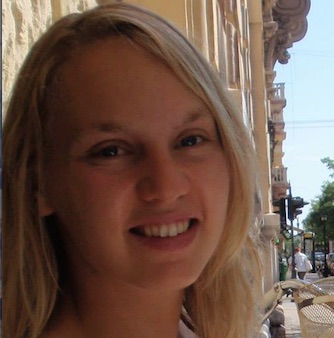# Seminar of Algebra

### Local and adelic Hecke algebra isomorphisms

Speaker:
Valentijn Karemaker (Utrecht University)
Email:
V.Z.Karemaker@uu.nl
Location:
Departamento de Álgebra
Date:
Fri, 22 apr 2016 12:30Valentijn Karemaker is a PhD student in algebraic number theory. Her research interests include Hecke algebras and their relation to anabelian geometry and Galois representations.

First, let $K$ and $L$ be number fields and let $G$ be a linear algebraic group over $Q$. Suppose that there is a topological group isomorphism of points on $G$ over the adeles of $K$ and $L$, respectively. We establish conditions on the group $G$, related to the structure of its Borel groups, under which $K$ and $L$ have isomorphic adele rings. As a corollary, we show that when $K$ and $L$ are Galois over $Q$, an isomorphism of Hecke algebras for $GL(n)$, which is an isometry in the $L_1$-norm implies that $K$ and $L$ are isomorphic as fields.

Secondly, let $K$ and $L$ be non-archimedean local fields of characteristic zero. Analogous results to the number fields case still hold. However, we show that the Hecke algebra for $GL(2)$ for any local field is Morita equivalent to the same complex algebra, determined by the Bernstein decomposition.